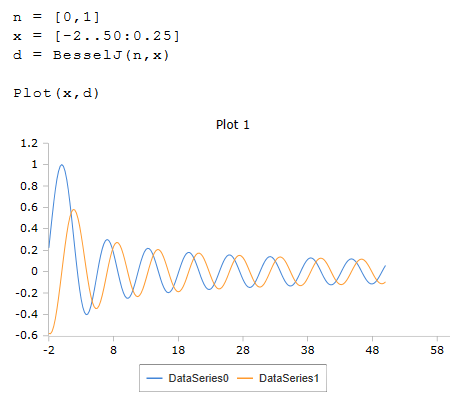# Besselj Function

Bessel function Jv(x) of the first kind

RedCrab Version 8.0

## Function Besselj

The function Besselj calculates the Bessel function Iv (x) of the first kind. The Bessel functions returns an oscillating behavior. A real or a complex number can be transferred as parameter x. A single value or a list can be passed.

Besselj (n, x)

## Function Besselje

The Besselje function differs from Besselj in that it uses the logarithmically scaled Bessel function for calculation A real or complex number, a single value or a list are also permitted as parameter x.

Besselje (n, x)

## Description

Single values or lists can be used as parameters n and x. The function is then calculated for each individual element of the list. The result is then a one-dimensional list, or a table if both parameters are lists.

If the real value of x is negative, the result can be a complex number.

### ExamplePlot of the Besselj function with ordinal numbers 0 and 1